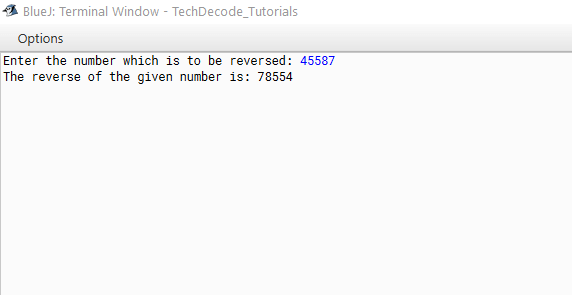# How to Reverse a Number in Java using recursion

Recursion is a problem-solving approach wherein the solution is built on the solutions to smaller instances of the same question. Iteration can usually manage such problems, but it requires identifying and storing the smaller instances at programming time. Recursion uses functions that call themselves from within their code to solve recursive problems. The method can be used to solve a range of problems, and recursion is a fundamental principle in computer programming. So today let us start with How to Reverse a Number in java using recursion.

## What’s The Approach?

• Import the java. util package into the class.
• Now within the main method create a new object of Scanner class,
• for example,` Scanner sc=new Scanner(System.in);`
• Use the Scanner class to get the input from the user.
• Print the Reversed number by calling the Recursive function with the parameter of the entered number
• End the main function
• Create a Recursive function with the Integer value as a parameter.
• If the number is less than 10 then print the same number
• Else, extract the last digit of the number by the modulus (%) operator and print that value
• Call the same function within that function only change the parameter by number/10.

Also Read: – Print Prime Factors of a Number in Java

### Java Program to Reverse a Number Using Recursion

```/*
* TechDecode Tutorials
*
* How to Reverse a number using Recursion in Java
*
*/

import java.util.*;
public class Reverse_Recursion
{

public static void main(String args[])
{
Scanner sc = new Scanner(System.in);
System.out.print("Enter the number which is to be reversed: ");
// Taking the input from user
int num = sc.nextInt();
System.out.print("The reverse of the given number is: " );
//calling the method to get the value of reversed number
Rev_Num(num);

}
// The recursive Function
public static void Rev_Num(int num)
{
// if the number is less than 10 then the reversed value is same as that number
if (num < 10)
{
//Printing the 1st digit of the entered number at the last when num value is less than 10
System.out.print(num);
return;
}
else
{
// extracting the last digit of the entered number
System.out.print(num % 10);
//calling the same fucntion itself by changing the value of num to num/10.
Rev_Num(num/10);
}
}

}```

`Input: 45587`

`Output: 78554`